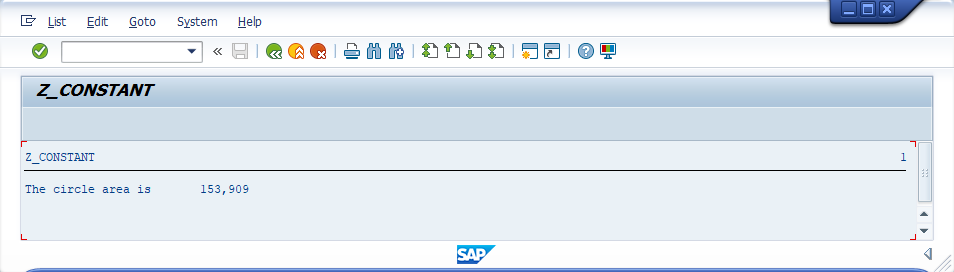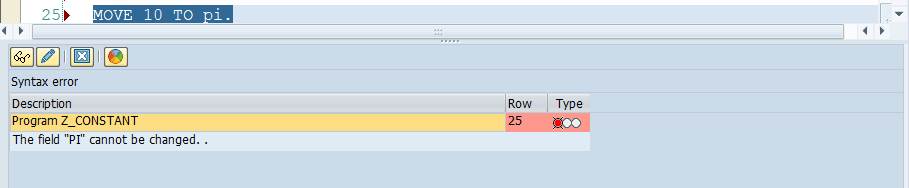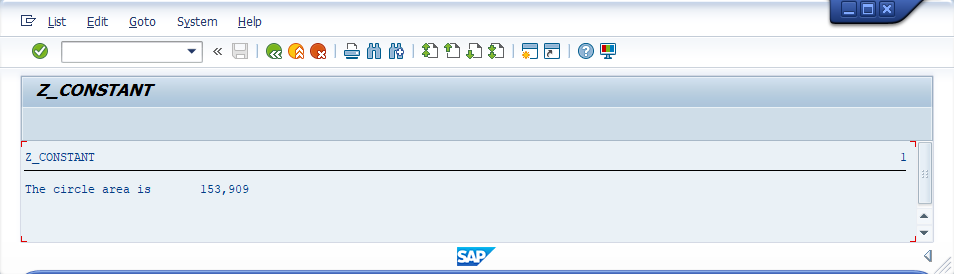###### Summary -

In this topic, we described about the below sections -

## What is Constant?

Constants are named objects that are used to store a fixed value and the value is fixed during program execution from begining to ending. The difference between constants and variables are, variables value can change any time however the constant value is fixed and can't be changed.

Constants are similar to variables and allows to store data under a particular name within the memory area of a program. Constants must have a VALUE clause coded and value should be assigned during the declaration itself. Constants can't be changed during the execution of the program. If tries to change the value of a constant, a syntax error or runtime error gets occured.

CONSTANTS keyword used to declare constants. Constants declared in class or interface are static variables of that class or interface.

Based on the place the variables declared within the program (like functions, classes, etc), the same rules of visibility apply to constants as apply to the visibility of data types.

#### Syntax -

``CONSTANTS {constant-name} TYPE {data-type} VALUE fixed-value.``

• constant-name - Specifies the constant name.
• data-type - Specifies the data type.
• fixed-value - Specifies the constant value.
Note! VALUE clause is mandatory during the constant declaration to assign a value.

The syntax of the CONSTANTS statement is same as the DATA statement with the following exceptions -

• VALUE addition is mandatory in the CONSTANTS statement and the value specified in the VALUE addition can't be changed during the execution of the program.
• Constants cannot define for strings, references, internal tables, or structures containing internal tables.

## Constant Types -

Constants are divided into three types based on it usage and those are -

• Elementary constants
• Complex constants
• Reference constants

### Elementary constants -

If a single variable is declared as constant, those called as elementary constants.

#### Syntax -

``````CONSTANTS {constant-name}
TYPE {data-type}
VALUE [constant-value|IS INITIAL].
CONSTANTS {constant-name}(length)
TYPE {data-type}
VALUE [constant-value|IS INITIAL].``````

#### Example -

Calculate the area of the circle (Area = π r²) when radius(r) is 7.

#### Code -

``````*&---------------------------------------------------------------------*
*& Report  Z_CONSTANT
*&---------------------------------------------------------------------*
*& Program Written by TUTORIALSCAMPUS
*&---------------------------------------------------------------------*

REPORT  Z_CONSTANT.

* Declaring a constant pi with a value 3.141.
CONSTANTS Pi TYPE P LENGTH 5 DECIMALS 3 VALUE '3.141'.

* Declaring variables for radius and area
lv_area   TYPE P LENGTH 6 DECIMALS 3.

* Assigning 7 as a circle radius

* calculating circle area with formula (A = πr²)

* Displaying the area after calculation.
WRITE: 'The circle area is ', lv_area.``````

#### Output -#### Explaining Example -

In the above example, each and every statement is preceeded with a comment to explain about the statement. Go through them to get clear understanding of example code.

Coming to output, lv_radius initialized with 7 and pi constant initialized with a value 3.141. So as per the formula(A = πr²), area calculated and the 153.909 displayed as a result.

Warning! As specified earlier, the constant value should not be changed in the program. If you are trying to change the value of constant, syntactical error gets occured.

For getting the error, we have been added the below code at the end of the above program.
``MOVE 10 TO pi.``

Th syntactical error gets occured is -### Complex constants -

Complex constants are group of elementary constants combined together.

#### Syntax -

``````CONSTANTS: BEGIN OF complex-constant-name,
…
END OF complex-constant-name.``````

#### Example -

Declare a complex constants and usage of complex constants in the program.

#### Code -

``````*&---------------------------------------------------------------------*
*& Report  Z_CONSTANT
*&---------------------------------------------------------------------*
*& Program Written by TUTORIALSCAMPUS
*&---------------------------------------------------------------------*

REPORT  Z_CONSTANT.

* Declaring a complex constant mc with Pi, sqrtof2 and piby2.
CONSTANTS: BEGIN OF mc,
Pi TYPE P LENGTH 5 DECIMALS 3 VALUE '3.141',
Sqrtof2 TYPE P LENGTH 5 DECIMALS 3 VALUE '1.414',
Piby2 TYPE P LENGTH 5 DECIMALS 3 VALUE '1.571',
END OF mc.

* Declaring variables for radius and area
lv_area   TYPE P LENGTH 6 DECIMALS 3.

* Assigning 7 as a circle radius

* calculating circle area with formula (A = πr²)

* Displaying the area after calculation.
WRITE: 'The circle area is ', lv_area.``````

#### Output -#### Explaining Example -

In the above example, each and every statement is preceeded with a comment to explain about the statement. Go through them to get clear understanding of example code.

Coming to output, lv_radius initialized with 7 and pi is a constant and also part of the complex constant mc. So the pi is reffered as mc-pi and initialized with a value 3.141. So as per the formula(A = πr²), area calculated and the 153.909 displayed as a result.

### Reference constants -

Reference constants are the references to the constants. Reference constants are used in comparisons and while passing data to procedures.

Reference constants are similar to the reference variable and the syntax as follows -

#### Syntax -

``CONSTANTS constant_pointer TYPE REF TO object_name VALUE IS INITIAL.``